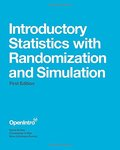## Open Textbooks#### Title

Introductory Statistics with Randomization and Simulation - First Edition

#### Contributors

David Diez - Author / Christopher Barr - Author / Mine Çetinkaya-Rundel - Author

#### Description

We hope readers will take away three ideas from this book in addition to forming a foundation of statistical thinking and methods. (1) Statistics is an applied field with a wide range of practical applications. (2) You don't have to be a math guru to learn from interesting/ real data. (3) Data are messy/ and statistical tools are imperfect. However/ when you understand the strengths and weaknesses of these tools/ you can use them to learn interesting things about the world. Textbook overview The chapters of this book are as follows: 1. Introduction to data. Data structures/ variables/ summaries/ graphics/ and basic data collection techniques. 2. Foundations for inference. Case studies are used to introduce the ideas of statistical inference with randomization and simulations. The content leads into the standard parametric framework/ with techniques reinforced in the subsequent chapters.1 It is also possible to begin with this chapter and introduce tools from Chapter 1 as they are needed. 3. Inference for categorical data. Inference for proportions using the normal and chi-square distributions/ as well as simulation and randomization techniques. 4. Inference for numerical data. Inference for one or two sample means using the t distribution/ and also comparisons of many means using ANOVA. A special section for bootstrapping is provided at the end of the chapter. 5. Introduction to linear regression. An introduction to regression with two variables. Most of this chapter could be covered immediately after Chapter 1. 6. Multiple and logistic regression. An introduction to multiple regression and logistic regression for an accelerated course. Appendix A. Probability. An introduction to probability is provided as an optional reference. Exercises and additional probability content may be found in Chapter 2 of OpenIntro Statistics at openintro.org. Instructor feedback suggests that probability/ if discussed/ is best introduced at the very start or very end of the course.

#### Subject 1

Mathematics - Applied

#### Subject 2

Mathematics - Applied

OpenIntro

#### Resources

Open Textbook Library